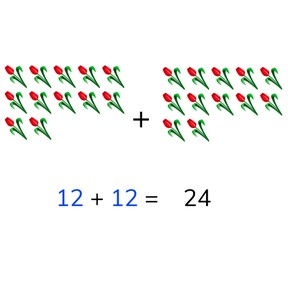I can add doubles to a total of 40.

No account needed.8,000 schools use Gynzy92,000 teachers use Gynzy1,600,000 students use Gynzy

## General

Students learn to add doubles to a total of 40.

2.OA.A.1

## Introduction

The interactive whiteboard shows four number sequences. Students determine what the missing numbers are and can check their answers by erasing the grey boxes.

## Instruction

Explain that when you have two numbers that are the same, they are doubles and that if you add the two, the total doubles. You can show this by counting the eggs. Use the images on the interactive whiteboard to support students in solving the addition problems. When showing the dice and balls, have students come up with the addition problem that matches the image before calculating to find the total. Next show students how to calculate the total without visual support. You can discuss the problems one by one. Students calculate the totals, and can drag the answers to the correct addition problems.

To check that students understand adding doubles to 40, you can ask the following questions:
- What do you do when you add doubles?
- What is the total of 16+16?

## Quiz

Students first practice finding the total of doubles by selecting from multiple choice answers. Then they must drag the answer to the addition problem, and finally they must write in the total themselves.

## Conclusion

Repeat the goal and discuss why it is useful to be able to add doubles. The interactive whiteboard shows three addition problems. Students must determine which of the three given answers match the given sums. Have students explain their thinking.

## Teaching tips

For students who have difficulty with this goal, they can be supported by using blocks or manipulatives. They can make groups representing each number and then count the objects together.

## Instruction materials

Optional: blocks or manipulatives

### The online teaching platform for interactive whiteboards and displays in schools

• Save time building lessons

• Manage the classroom more efficiently

• Increase student engagement